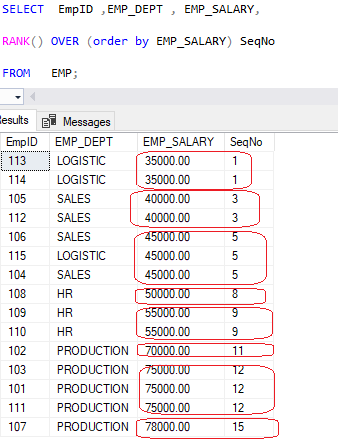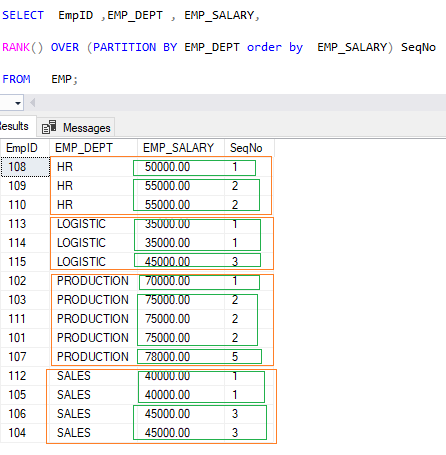Home » SQL RANK()

# SQL RANK()The RANK()  function is a Ranking or window function that is used to assign a rank to each row in the partition of a result set.

The rows within a partition that have the same values will have the same rank.

The rank of the first row within a partition is one. The rank of a row is assigned by one plus the number of ranks that come before it.

The same column values receive the same ranks. When multiple rows share the same rank, the rank of the next row is not consecutive.

SYNTAX

```Select RANK() OVER (

[PARTITION BY columnnames, ... ]

ORDER BY columnname [ASC | DESC]

) , Col1 , Col2 ,..

from tablename```
PARTITION BY

The PARTITION BY clause divides the result set into partitions.

ORDER BY

The ORDER BY clause defines the logical order of the rows within each partition of the result set.

RANK()

RANK()  function is operated on the rows of each partition and re-initialized when crossing each partition boundary.

Lets look at an example of RANK() in SQL

```SELECT EmpID ,EMP_DEPT , EMP_SALARY,

RANK() OVER (order by EMP_SALARY) SeqNo

FROM EMP;

```As we can see, the first and second rows share the same rank because they have the same value. The third row gets the rank 3 because the RANK() function skips the rank 2.

#### RANK() Function with PARTITION BY

To assign the Rank to employee by partitioning the department based on salary , Using Rank() function with Partition By is given below.

```SELECT EmpID ,EMP_DEPT , EMP_SALARY,

RANK() OVER (PARTITION BY EMP_DEPT order by EMP_SALARY) SeqNo

FROM EMP;```